# Lines

Here you will learn about lines, including what they are, what they are made of and different ways to classify sets of lines.

Students will first learn about lines as part of geometry in 4 th grade.

## What are lines?

In geometry, Lines are one-dimensional figures that are straight and extend forever in opposite directions.

For example,

Lines can be broken down into many different parts. One part is a line segment.

A line segment is part of a line that has a beginning and an end point.

For example,

Line segments are seen in many common 2D shapes and real world items.

For example,

Each side of a triangle is made up of line segments – parts of straight lines.

Step-by-step guide: Line segments

A line can also be broken down into a ray. A ray is a part of a line that has one endpoint, but extends forever in the other direction.

For example,

Rays are also seen in geometric angles, inequality graphs and real world items.

For example,

Angle F is made up of two rays → ray FY and ray FO .

Step-by-step guide: Ray math

When more than one line is present, there is a relationship. There are two types of line relationships: lines that intersect (cross) or lines that never intersect. When lines are always the same distance apart and never intersect, they are parallel lines.

For example,

Each side of a rectangle is made of a line segment that is part of a line. The opposite sides of a rectangle are parallel.

There are also many examples of parallel lines in real life.

For example,

The lines that pass by the legs of a table are parallel.

Step-by-step guide: Parallel lines

When lines intersect and form a 90 -degree angle, they are perpendicular lines.

Each side of a rectangle is perpendicular to the adjacent sides (the sides that touch). This is why the corners of a rectangle always form right angles.

There are also many examples of perpendicular lines in real life.

For example,

A table’s legs and the flat tabletop are perpendicular to each other. A 90^{\circ} angle is formed where the legs and the tabletop meet.

Step-by-step guide: Perpendicular lines

### What are lines?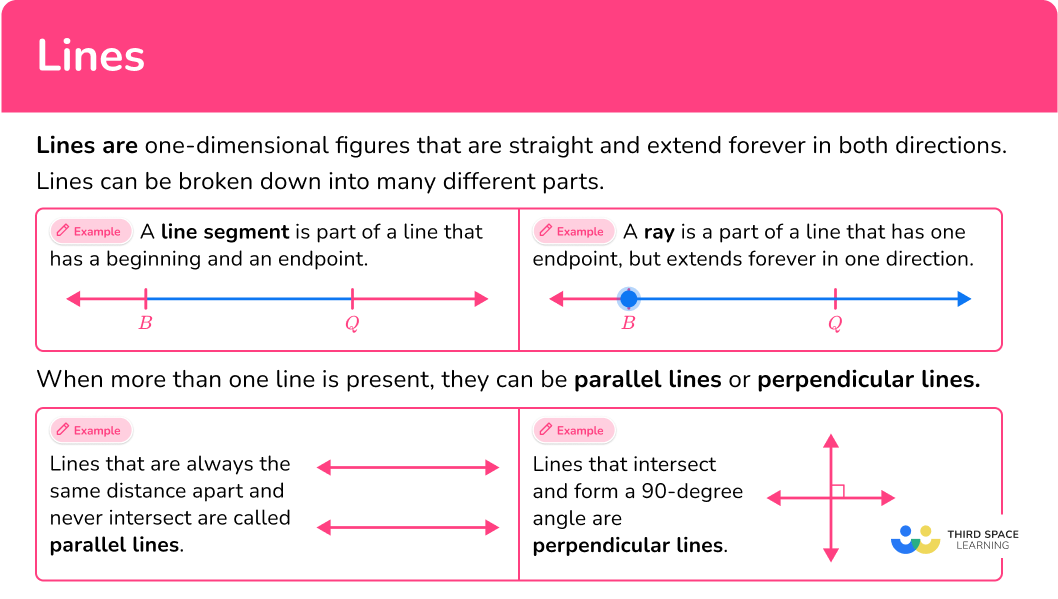## Common Core State Standards

How does this relate to 4 th grade math?

• 4th Grade – Geometry (4.G.A.1)
Draw points, lines, line segments, rays, angles (right, acute, obtuse), and perpendicular and parallel lines. Identify these in two-dimensional figures.

## How to identify lines, rays and line segments

In order to identify lines, rays and line segments:

1. Look at each end and decide if it is a point or never ending.
2. Name the figure as a line, line segment, or ray.
3. If there is more than one figure, decide if it is an example of parallel lines or perpendicular lines.

## Lines examples

### Example 1: one dimensional figure

What type of figure is shown?

1. Look at each end and decide if it is a point or never ending.

The left end is a point and the right end is never ending.

2Name the figure as a line, line segment, or ray.

The figure is a ray.

### Example 2: one dimensional figure

What type of figure is shown?

Neither side of the figure has an endpoint. Both sides are never ending.

The figure is a line.

### Example 3: one dimensional figure

Highlight the line, line segment and ray shown in the figure below

The left end is never ending and the right end is never ending. There are two points, T and R.

Because there are two points, this figure can represent all three. Let’s look at each.

The figure shows line TR, which goes in each direction and never ends:

The figure shows line segment TR, which has two endpoints:

The figure shows ray TR and ray RT, which each have one endpoint, and the other end goes on forever:

### Example 4: lines in a 2-dimensional shape

Highlight any sets of parallel lines or perpendicular lines in the compound shape (a rectangle and a right triangle).

Each vertex is a point, and no part of the shape is never ending.

Each side of the shape is made of a line segment that is part of a line. These lines cross at the vertices of the shape (which are also the endpoints of the line segments).

The shape has parallel lines and perpendicular lines. Let’s look at each.

Parallel lines are lines that are the same distance apart and never touch.

The blue lines are parallel to each other and the green lines are parallel to each other.

Since the shape is made up of a rectangle and a right triangle, the two left corners and the far right corner are right angles ( 90^{\circ} ).

Where the rectangle and triangle meet, they also appear to form right angles. This means the intersecting lines at these vertices are perpendicular.

There are five sets of perpendicular lines created by shape.

### Example 5: identifying parallel lines and perpendicular lines

Identify sets of lines that appear to be parallel or perpendicular.

Both sides of each figure are never ending.

The figures are identified as lines.

Parallel lines are lines that are the same distance apart and never touch.

Line X and Line B appear to be parallel.

Perpendicular lines are intersecting lines that cross and form four right angles ( 90^{\circ} ).

Line A and Line Y appear to be perpendicular.

### Example 6: lines in the real world

Find a set of parallel lines and perpendicular lines.

The sides of the picture frame have endpoints.

Each side of the shape is made of a line segment that is part of a line.

Parallel lines are lines that are the same distance apart and never touch.

The blue lines are parallel to each other and the green lines are parallel to each other.

The picture frame is made up of two rectangles. Each side of a rectangle is perpendicular to the adjacent sides (the sides that touch). This is why the corners of a rectangle always form right angles.

The lines that form the corners are perpendicular.

### Teaching tips for lines

• Worksheets that provide a variety of figures (lines, line segments, rays) can be a good way to learn how to identify them. However, it is also important to teach students to look for these figures in the real world.

• Encourage students to go beyond just rotely solving practice problems to also creating each type of figure, and require them to explain why a figure is classified the way it is.

• Challenge students to add new lines to a given example of parallel or perpendicular lines. For example, given a set of parallel lines, ask students to draw a line that is not parallel to the two lines, a line that is parallel to the two lines, and a line that creates a set of perpendicular lines.

• To incorporate art, ask students to create a design that includes all the types of figures covered on this page. For an extension, they can compare their art to a partner’s and see who has more of each type of figure within their creation.

### Easy mistakes to make

• Confusing parallel and perpendicular lines
Since they are often introduced together, in the beginning, it is easy for students to confuse these two vocabulary words. With enough practice and real world application, students will learn the difference.

• Thinking parallel lines have to be vertical lines or horizontal lines
Parallel lines can be in any orientation as long as they are lines that are the same distance apart and never intersect.
For example,

• Thinking lines and line segments are synonyms
Lines and line segments are not the same thing. Line segments have endpoints, whereas lines go on forever.

### Practice lines questions

1) What type of figure is shown below?lineline segmentrayparallel linesThe figure has two endpoints and is part of a line, so it is a line segment.

2) What is the best classification for the figure below?lineline segmentraypointThe figure has no endpoints and goes on forever in opposite directions, so it is a line – which is why it is commonly referred to as a number line.

3) Which choice shows rays in the shape?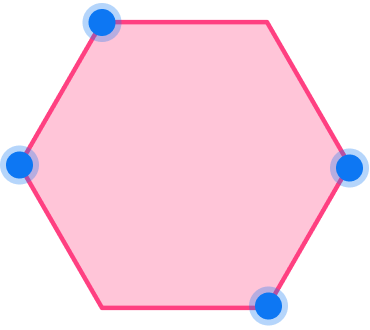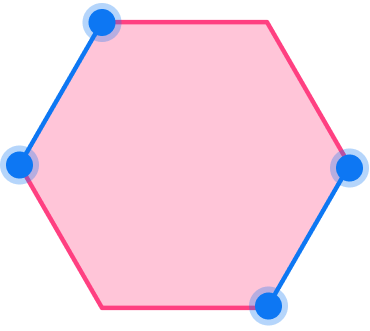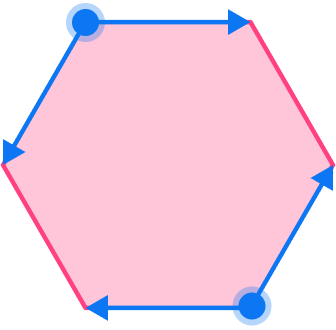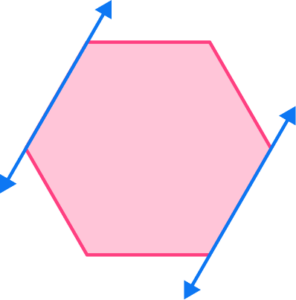Rays have one endpoint and an opposite side that goes on forever. Two rays with a common endpoint form an angle.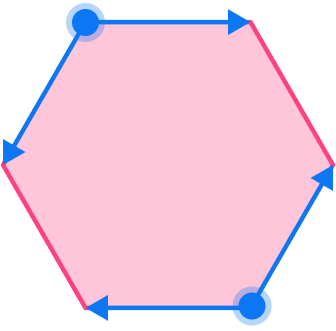These two angles show four rays that are in the shape.

4) Complete the sentence:
The part of the New York state line shown in pink is an example of a(n)…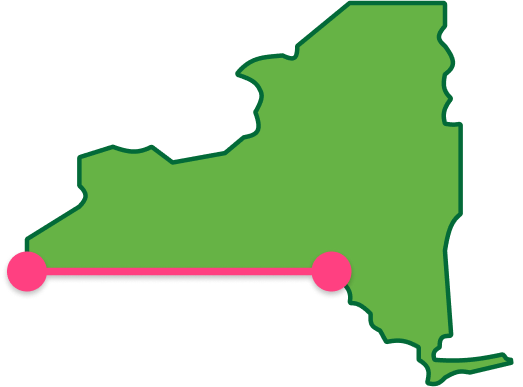anglelinerayline segmentThe figure has two endpoints and is part of a line, so it is a line segment.

5) Which choice shows parallel lines formed by the sides of the shape?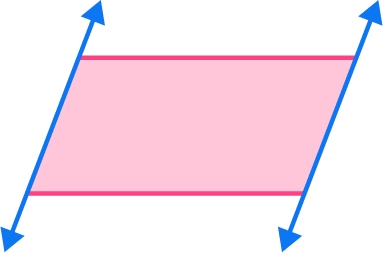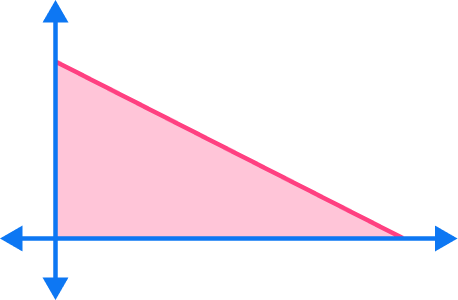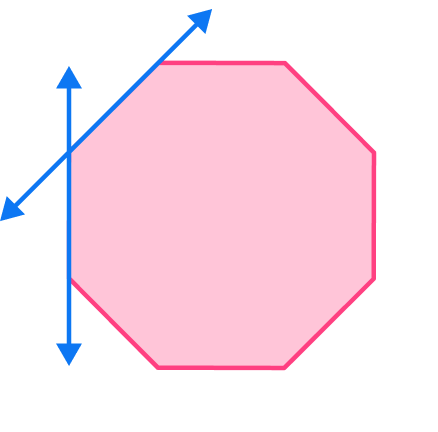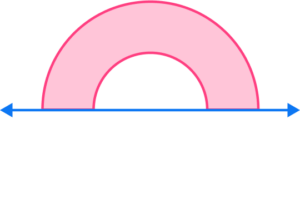Parallel lines are lines that are always the same distance apart and never intersect.The sides of a parallelogram are parallel.

6) Which lines are perpendicular?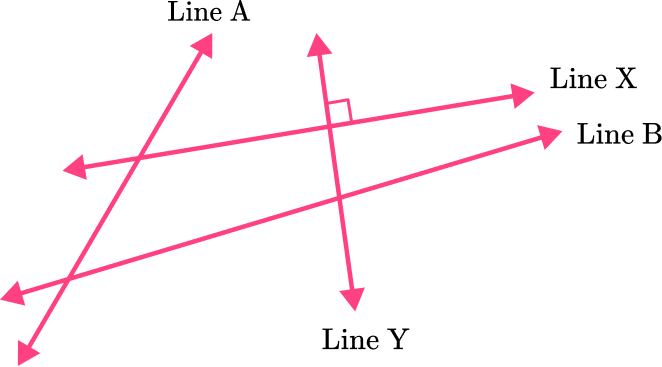Line Y and Line BLine A and Line XLine Y and Line XLine A and Line BPerpendicular lines are intersecting lines that cross and form four right angles (90^{\circ}).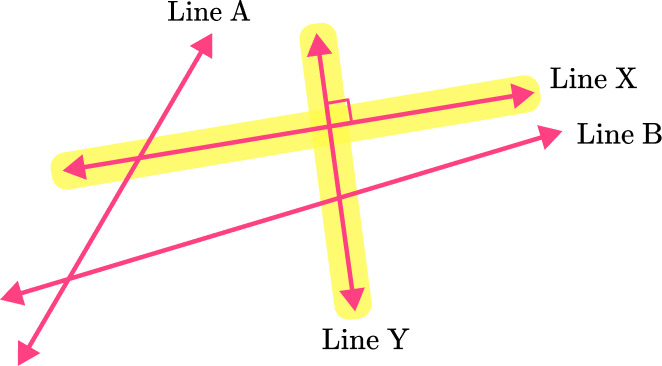Line Y and Line X are perpendicular lines.

## Lines FAQs

What is a line?

A set of points that go in opposite directions on a straight path.

What is a polygon?

A general definition for a polygon is a straight sided, closed figure. Shapes like triangles, squares, and octagons are polygons. Shapes like a circle or a ray are not polygons.

What are collinear points?

All points on a line are collinear – meaning they are on the same line.

What is a transversal line?

A line that passes through at least two other lines at specific points.

Can line segments have fractions as the length of the line?

Yes, a line segment can have a fractional length. The measurement does not have to be equal to a whole number.

## Still stuck?

At Third Space Learning, we specialize in helping teachers and school leaders to provide personalized math support for more of their students through high-quality, online one-on-one math tutoring delivered by subject experts.

Each week, our tutors support thousands of students who are at risk of not meeting their grade-level expectations, and help accelerate their progress and boost their confidence.